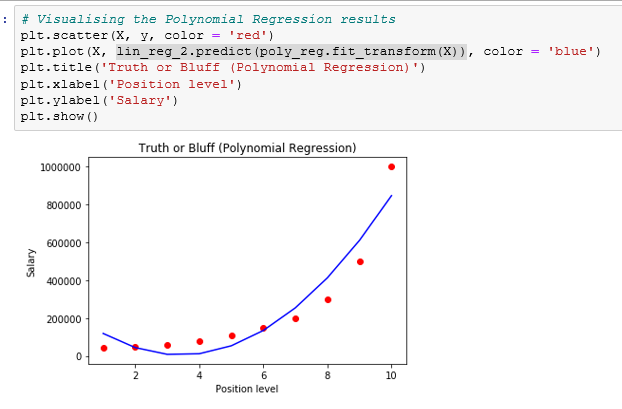# Cruising through the basics of Polynomial Regression

Source: Deep Learning on Medium# Cruising through the basics of Polynomial Regression

Polynomial Regression is a form of linear regression in which the relationship between the independent variable x and dependent variable y is modeled as an nth degree polynomial. Polynomial regression fits a nonlinear relationship between the value of x and the corresponding conditional mean of y, denoted E(y |x). I assume that the readers have a clear understanding of Linear regression. I wont dive deep into mathematics and will try to give you a hands-on experience of polynomial regression in Python.

Let us take the basic data set to explain the concept.